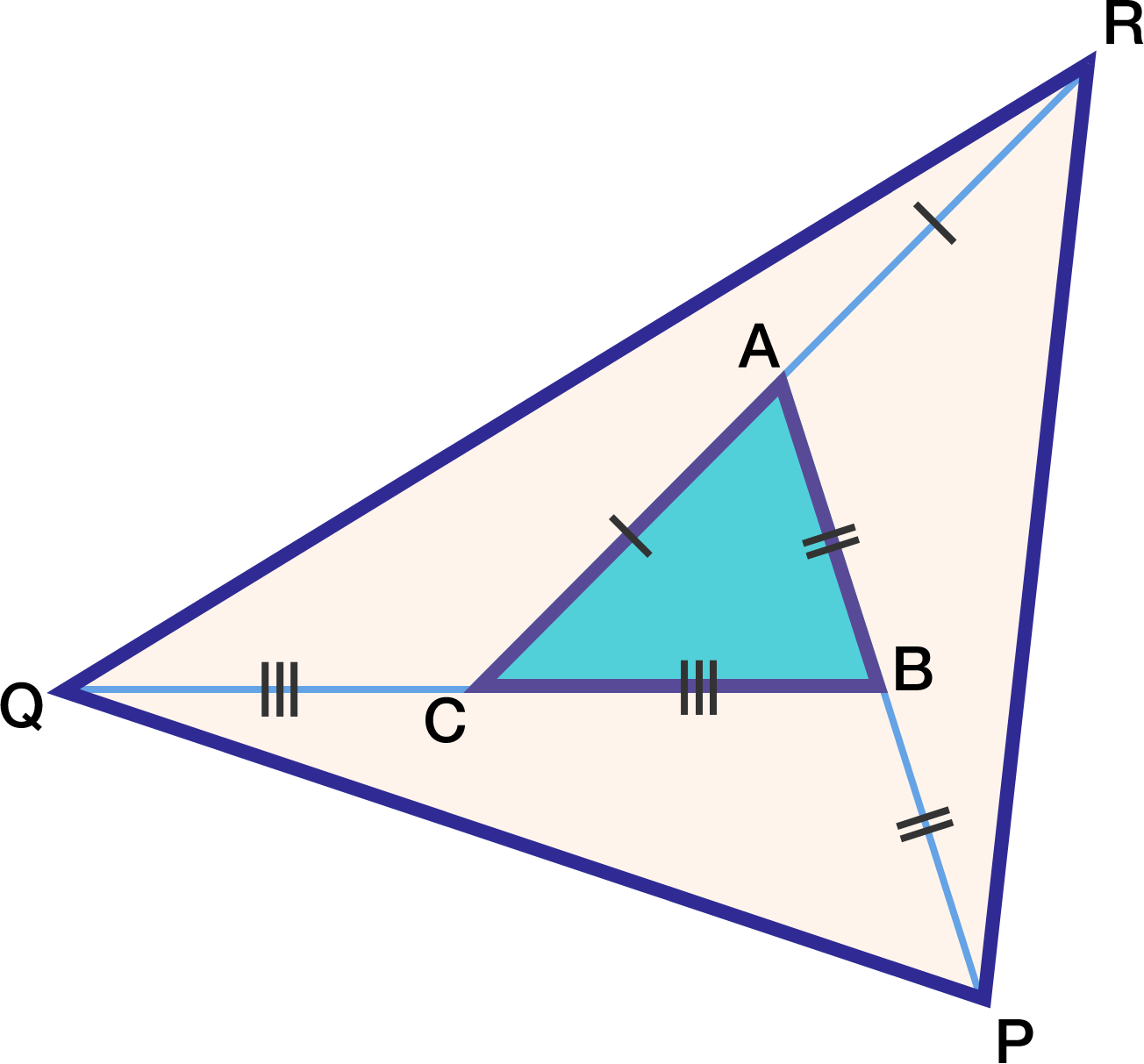Geometry Level 3Each side of triangle $ABC$ is extended such that $AB=BP, BC=CQ, CA=AR,$ as shown in the diagram above.

If the area of triangle $ABC$ is 10, then what is the area of triangle $PQR?$

×